Thermodynamics and Propulsion
Subsections

# 1.2 Definitions and Fundamental Ideas of Thermodynamics

As with all sciences, thermodynamics is concerned with the mathematical modeling of the real world. In order that the mathematical deductions are consistent, we need some precise definitions of the basic concepts. The following is a discussion of some of the concepts we will need. Several of these will be further amplified in the lectures and in other handouts. If you need additional information or examples concerning these topics, they are described clearly and in-depth in (SB&VW). They are also covered, although in a less detailed manner, in Chapters 1 and 2 of the book by Van Ness.

## 1.2.1 The Continuum Model

Matter may be described at a molecular (or microscopic) level using the techniques of statistical mechanics and kinetic theory. For engineering purposes, however, we want averaged'' information, i.e., a macroscopic, not a microscopic, description. There are two reasons for this. First, a microscopic description of an engineering device may produce too much information to manage. For example,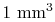of air at standard temperature and pressure contains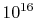molecules (VW, S & B:2.2), each of which has a position and a velocity. Typical engineering applications involve more thanmolecules. Second, and more importantly, microscopic positions and velocities are generally not useful for determining how macroscopic systems will act or react unless, for instance, their total effect is integrated. We therefore neglect the fact that real substances are composed of discrete molecules and model matter from the start as a smoothed-out continuum. The information we have about a continuum represents the microscopic information averaged over a volume. Classical thermodynamics is concerned only with continua.

## 1.2.2 The Concept of a System''

A thermodynamic system is a quantity of matter of fixed identity, around which we can draw a boundary (see Figure 1.3 for an example). The boundaries may be fixed or moveable. Work or heat can be transferred across the system boundary. Everything outside the boundary is the surroundings.

When working with devices such as engines it is often useful to define the system to be an identifiable volume with flow in and out. This is termed a control volume. An example is shown in Figure 1.5.

A closed system is a special class of system with boundaries that matter cannot cross. Hence the principle of the conservation of mass is automatically satisfied whenever we employ a closed system analysis. This type of system is sometimes termed a control mass.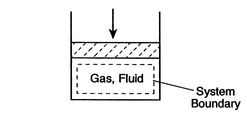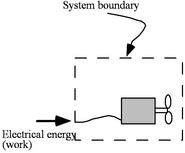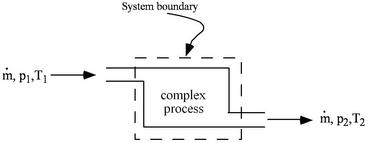## 1.2.3 The Concept of a State''

The thermodynamic state of a system is defined by specifying values of a set of measurable properties sufficient to determine all other properties. For fluid systems, typical properties are pressure, volume and temperature. More complex systems may require the specification of more unusual properties. As an example, the state of an electric battery requires the specification of the amount of electric charge it contains.

Properties may be extensive or intensive. Extensive properties are additive. Thus, if the system is divided into a number of sub-systems, the value of the property for the whole system is equal to the sum of the values for the parts. Volume is an extensive property. Intensive properties do not depend on the quantity of matter present. Temperature and pressure are intensive properties.

Specific properties are extensive properties per unit mass and are denoted by lower case letters. For example: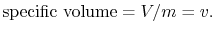Specific properties are intensive because they do not depend on the mass of the system.

The properties of a simple system are uniform throughout. In general, however, the properties of a system can vary from point to point. We can usually analyze a general system by sub-dividing it (either conceptually or in practice) into a number of simple systems in each of which the properties are assumed to be uniform.

It is important to note that properties describe states only when the system is in equilibrium.

Muddy Points

Specific properties (MP 1.1)

What is the difference between extensive and intensive properties? (MP 1.2)

## 1.2.4 The Concept of Equilibrium''

The state of a system in which properties have definite, unchanged values as long as external conditions are unchanged is called an equilibrium state.

 [Mechanical Equilibrium]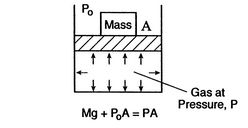[Thermal Equilibrium]A system in thermodynamic equilibrium satisfies:

1. mechanical equilibrium (no unbalanced forces)
2. thermal equilibrium (no temperature differences)
3. chemical equilibrium.

## 1.2.5 The Concept of a Process''

If the state of a system changes, then it is undergoing a process. The succession of states through which the system passes defines the path of the process. If, at the end of the process, the properties have returned to their original values, the system has undergone a cyclic process or a cycle. Note that even if a system has returned to its original state and completed a cycle, the state of the surroundings may have changed.

## 1.2.6 Quasi-Equilibrium Processes

We are often interested in charting thermodynamic processes between states on thermodynamic coordinates. Recall from the end of Section 1.2.3, however, that properties define a state only when a system is in equilibrium. If a process involves finite, unbalanced forces, the system can pass through non-equilibrium states, which we cannot treat. An extremely useful idealization, however, is that only infinitesimal'' unbalanced forces exist, so that the process can be viewed as taking place in a series of quasi-equilibrium'' states. (The term quasi can be taken to mean as if;'' you will see it used in a number of contexts such as quasi-one-dimensional, quasi-steady, etc.) For this to be true the process must be slow in relation to the time needed for the system to come to equilibrium internally. For a gas at conditions of interest to us, a given molecule can undergo roughly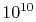molecular collisions per second, so that, if ten collisions are needed to come to equilibrium, the equilibration time is on the order of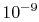seconds. This is generally much shorter than the time scales associated with the bulk properties of the flow (say the time needed for a fluid particle to move some significant fraction of the length of the device of interest). Over a large range of parameters, therefore, it is a very good approximation to view the thermodynamic processes as consisting of such a succession of equilibrium states, which we can chart. [VW, S& B: 2.3-2.4]

The figures below demonstrate the use of thermodynamics coordinates to plot isolines, lines along which a property is constant. They include constant temperature lines, or isotherms, on a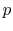-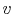diagram, constant volume lines, or isochors on a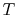-diagram, and constant pressure lines, or isobars, on a-diagram for an ideal gas.

Real substances may have phase changes (water to water vapor, or water to ice, for example), which we can also plot on thermodynamic coordinates. We will see such phase changes plotted and used for liquid-vapor power generation cycles in Chapter 8. A preview is given in Figure 1.15 at the end of this chapter.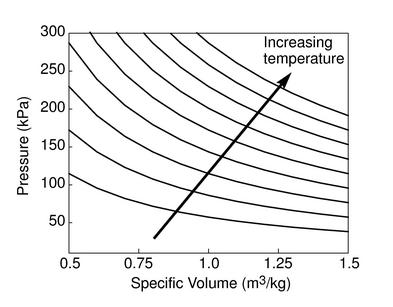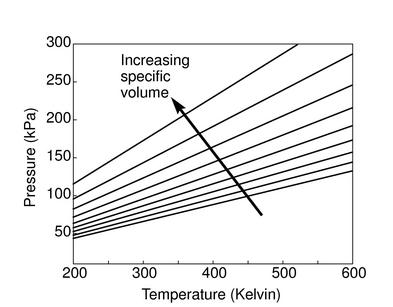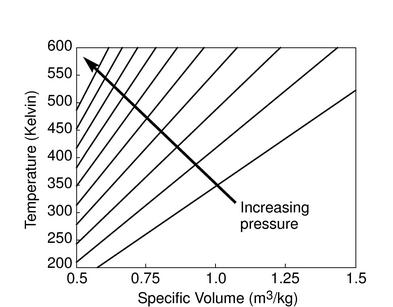## 1.2.7 Equations of state

It is an experimental fact that two properties are needed to define the state of any pure substance in equilibrium or undergoing a steady or quasi-steady process. [VW, S & B: 3.1, 3.3]. Thus for a simple compressible gas like air,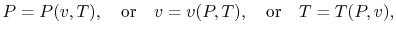whereis the volume per unit mass,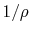. In words, if we knowandwe know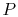, etc.

Any of these is equivalent to an equation, which is known as an equation of state. The equation of state for an ideal gas, which is a very good approximation to real gases at conditions that are typically of interest for aerospace applications1.2, iswhereis the volume per mol of gas and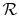is the Universal Gas Constant,''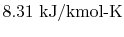.

A form of this equation which is more useful in fluid flow problems is obtained if we divide by the molecular weight,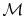: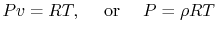where R is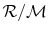, which has a different value for different gases due to the different molecular weights. For air at room conditions,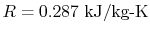.

Douglas Quattrochi 2006-08-06